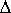QUESTION #605

# Is the law of conservation of energy violated for a short or long period of time and can it be experimentally observed?

In classical mechanics (in the sense of non-quantum) physics, there is no mechanism to allow for non-conservation for energy. Since classical mechanics is pretty exact for macroscopic objects, there is never any macroscopic violation of conservation of energy. However, for small enough (quantum) systems, we have the Heisenberg uncertainty relation. The relation states:E xt >= h/(4) Which means that the uncertainty in energy times the uncertainty in time is greater than some very small number. Interpreted correctly, this means that it is possible to violate conservation of energy given you do it for a very short time, in other words, it is possible to "borrow" energy E from "nowhere", given you return it in a time period t, where t is given by t ~ h/E This "borrowing" of energy is so small that again by the uncertainty principle, it can never be directly observed. In other words, violation of conservation of energy can occur if and only if the violation can not be observed due to the uncertainty principle. However, there are "indirect" effects. One simple example could be the nuclear force. The longest range part of the nuclear force is mediated by exchange of pions (similar to the electromagnetic force being mediated by photons). However, these pions are "virtual", meaning that they can not be detected. They are just produced out of nowhere just like energy. The amount of energy you need to create a virtual pion is: E = mc2 where m is the mass of the pion, and c is the speed of light. Now, how long can we "borrow" this much energy? By our previous argument, the time will be: t ~ h/mc2 If this virtual pion moves as fast as possible, it will move at the speed of light. Then the distance it can travel is: d = ct ~ h/mc Of course, after traveling that distance, it would have to be absorbed by another particle (and thus mediate the force). Therefore d is the approximate range of the force. The amazing thing is, this gives a pretty good value for the range of the nuclear force! To sum it up, yes, conservation of energy can be violated, but nature makes sure it is always within the limits of uncertainty. In other words, the energy must be returned, and the books set straight pretty quickly. But, the fact that it can be violated is important, and although it can never be observed directly, it does have important consequences.